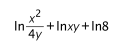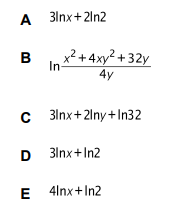# IMAT 2013 Q58 [Simplifying]

SimplifyUsing the Natural Logarithm laws:

1. \log _{a}x\cdot y = \log _{a}x + \log _{a}y
2. \log _{a}\frac{x}{y} = \log _{a}x - \log _{a}y
3. \log _{a} x^{m} = m \log _{a}x
4. \log _{a} \sqrt[n]{x} = \frac{1}{n} \log _{a}x

p.s. \ln =\log _{e}

\ln\frac{x^2}{4y} + \ln xy + \ln8 = (\ln x^2 - \ln 4y )+ (\ln x + \ln y )+ \ln 2^3
= (2\ln x -(\ln 4 + \ln y)) + \ln x +\ln y + 3\ln 2 = 2\ln x - \ln 2^2 - \ln y + \ln x + \ln y + 3\ln 2
=3\ln x - 2\ln 2 +3\ln 2 \to 3\ln x + \ln 2.

Which is the answer to the question. (D)

If more explanation is needed, please ask me further. I tried to simplify it as much as i could.